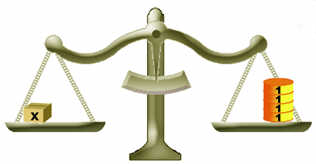Definition of

AlgebraAlgebra uses letters (like x or y) or other symbols in place of values, and then plays with them using special rules.

Example: x + 3 = 7
"x" is used in place of a value we don't know yet and is called the "unknown" or the "variable".

In this case the value of "x" can be found by subtracting 3 from both sides of the equal sign like this:

Start with: x + 3 = 7
Subtract 3 from both sides: x + 3 − 3 = 7 − 3
Calculate: x + 0 = 4
Answer: x = 4